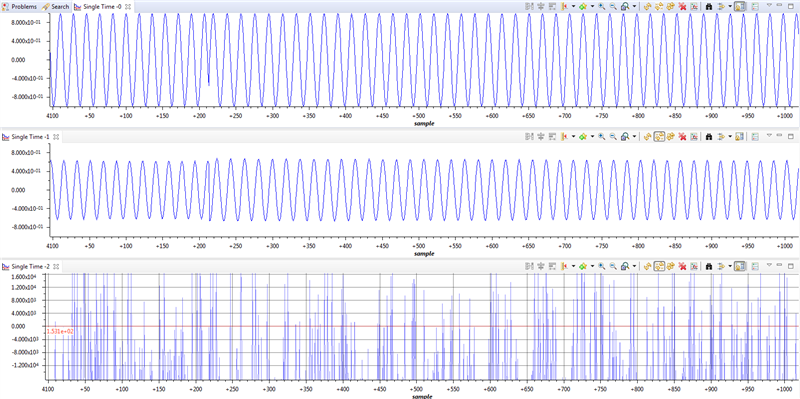# CMSIS DSP Biquad fixpoint error on Cortex M4

Hi

I have a problem by implementing a CMSIS Biquad lowpass filter an a Cortex M4 from TI.

The CMSIS FLOAT filter is working but not the fixpoint  Q15 one.

It's just a simple 2nd order (1 stage) filter. The coeffs are calculated with Matlab.

Did anybody tried this allready and see an error in here?

The chrip function generate a chirps sinus with an amplitude of +-1.

René

```// fs = 16000;
// fg = 1600 Hz, Butter
// [z,p,k] = butter(2,fg/fs,'low');
// sos2 = zp2sos(z,p,k);
// Hd = dfilt.df2tsos(sos2);
// fvtool(Hd,'Analysis','freq')
// coef = coeffs(Hd);
// coef.SOSMatrix
// ans = 0.0201    0.0402    0.0201    1.0000   -1.5610    0.6414

#define STAGES ((uint8_t)1)

// a11 und a12 müssen negiert werden
float fCoeffs[] = { 0.0200833659619093,  0.0401667319238186,  0.0200833659619093,    1.56101810932159,   -0.641351521015167 };
float32_t IIRstate[2*STAGES];

// a11 und a12 müssen negiert werden
//                             b10, 0, b11, b12,   a11,    a12
q15_t n16Coeffs[STAGES * 6] = {329, 0, 658, 329, 25576, -10508};
q15_t n16StateA[STAGES * 4];

float fFilterIn = chirp.calc_log();
fFilterOut1 = BiQuadFloat.filter(&fFilterIn);q15_t n16FilterIn = (q15_t)(fFilterIn * 16384.0f); // only scaled by Q14 for test

And this is what I get.

The first chart is the chirp signal (fFilterIn).

The 2nd is the output of the float filter (fFilterOut1).

The 3rd is the output of the fixpoint filter (n16FilterOutQ15).More questions in this forum

35 views
3 replies

126 views
3 replies

116 views
3 replies
Latest

534 views
3 replies
Latest